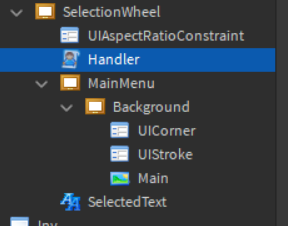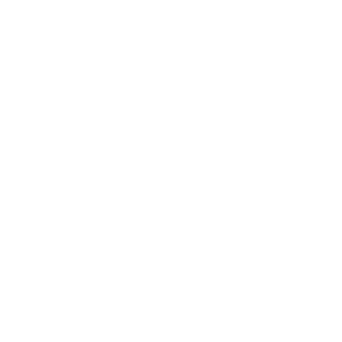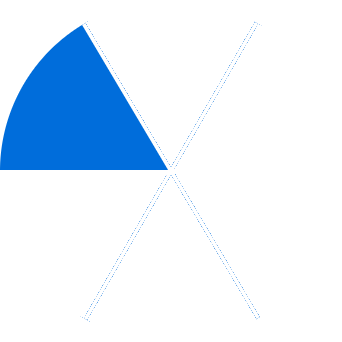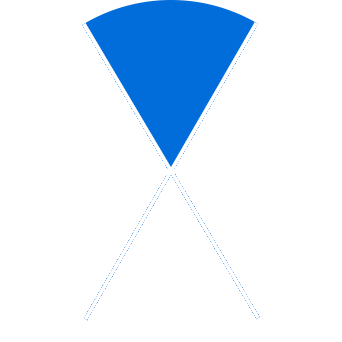# Check if mouse is hovering over a triangular UI area

Hey there, I am currently trying to create a selection wheel UI for a main menu for my game.
This is what it looks like at the moment:Currently the handler script is empty, as I have no clue where to even start on this.
Basically, I want to detect if he mouse is in once of the areas, be it the top triangle, the bottom or whatever. If it is in one of those areas, the image would either be replaced by another image which has the currently selected triangle ‘selected’, or to rotate the image to where the mouse is, but it would need to snap somehow, and I once again have no clue how do to that.

If you are confused by the first option I proposed, here are a couple of images:Any help would be greatly appreciated, as I have not been able to find anything that can help me on this so far.

Thank you

1 Like

Do you have images of all the possible cases of the blue triangle?

1 Like

Never mind, I figured it out myself, here is the script if anyone is interested in seeing how I did it:

``````local rs = game:GetService("RunService")

local player = game.Players.LocalPlayer
local mouse = player:GetMouse()

rs.Stepped:Connect(function()
local frameCenter = frame.AbsolutePosition + (frame.AbsoluteSize/2)
local x = math.atan2(mouse.Y - frameCenter.Y, mouse.X - frameCenter.X)
--print(x)
if x < -1.1 and x > -2 then
frame.Rotation = 0
elseif x < -2.1 and x > -3 then
frame.Rotation = -60
elseif x < 3.1 and x > 2.1 then
frame.Rotation = -120
elseif x < 2 and x > 1 then
frame.Rotation = -180
elseif x < 1 and x > 0.2 then
frame.Rotation = -240
elseif x < 0 and x > -1 then
frame.Rotation = -300
end
end)
``````

Basically, I printed the value of x repeatedly and got the values of where each segment is between. I then put that into notepad, stopped the game, and just changed the rotation accordingly.

I know it isn’t that efficient, if anyone wants to edit it then go ahead!

1 Like